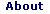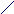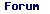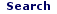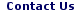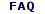Fri - 02 Jun 2023

WELCOME TO RFCABLES.ORG

## reflection coefficient Calculator:

The reflection coefficient is the ratio of reflected wave to incident wave at point of reflection. This value varies from -1 (for short load) to +1 (for open load), and becomes 0 for matched impedance load.
Formala is: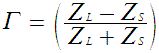where ZS is the impedance toward the source,
ZL is the impedance toward the load.

 ZS(impedance toward the source)= ZL(impedance toward the load)=The reflection coefficient=

## return loss Calculator:

This is the dB value of absolute reflection coefficient.
This loss value becomes 0 for 100% reflection and becomes infinite for ideal connection.
Formula is::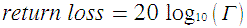[dB]

 reflection coefficient(Γ)=[dB] return loss =

## return loss Calculator:(by using VSWR)

Formula is::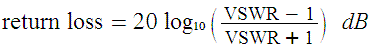VSWR=return loss =

## VSWR Calculator:

This is the ratio of maximum voltage to minimum voltage in standing wave pattern.
It varies from 1 to (plus) infinity.
Formula is::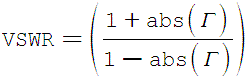reflection coefficient(Γ)=VSWR =

## VSWR Calculator:(by using RL)

 return loss(RL)=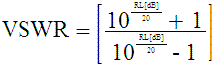VSWR =